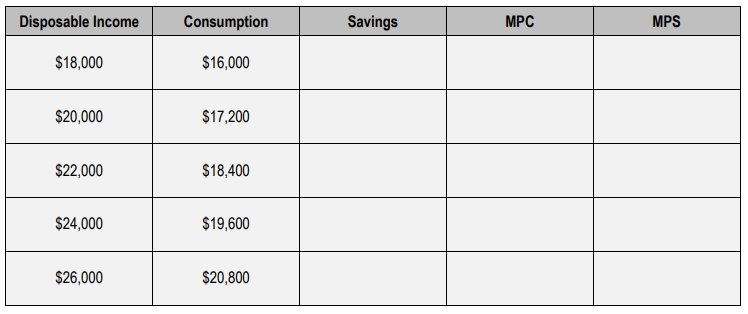Start typing, then use the up and down aroows to select an option from the list.

# Macroeconomics

Learn the toughest concepts covered in your Macroeconomics class with step-by-step video tutorials and practice problems.

Income and Consumption
1

### concept

Consumption Function (Consumption Schedule)9m
Play a video:
0
2

### concept

Marginal Propensity to Consume and Save4m
Play a video:
0
3

### concept

1 = MPC + MPS2m
Play a video:
0
4

### concept

Equation of the Consumption Function2m
Play a video:
0
5
Problem

Calculate the Marginal Propensity to Consume and the Marginal Propensity to Save using the following table:6
Problem

If the Keynesian consumption function is C = 10 + 0.8 Yd then, if disposable income is \$1000, what is amount of total consumption?

7
Problem

If the Keynesian consumption function is C = 10 + 0.8 Yd then, when disposable income is \$1000, what is the marginal propensity to consume?

8
Problem

An increase in the marginal propensity to consume will: